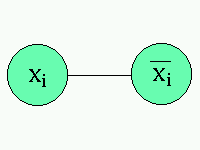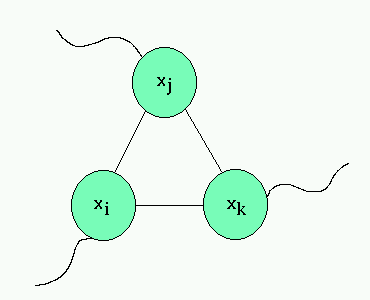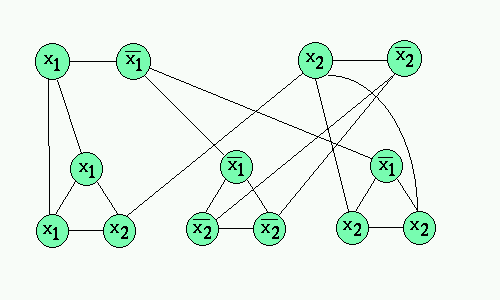Introduction

Complexity
The Art-Gallery

The Proofs
Conclusions

References

# A Sample Proof of NP-Completeness

The following is the proof that the problem VERTEX COVER is NP-complete. This particular proof was chosen because it reduces 3SAT to VERTEX COVER and involves the transformation of a boolean formula to something geometrical. This is similar to what will be done for the two art gallery proofs.

This proof closely follows the one in "Computers and Intractability" by Garey and Johnson. A similar proof can be found in "Introduction to the Theory of Computation" by Sisper as this NP-completeness proof is quite basic.

Let us begin by defining the problems. First,
3-SATISFIABILITY (3SAT)
Instance: Set U of variables, a collection C of clauses over U such that each clause c in C has size exactly 3.
Question: Is there a truth assignment for U satisfying C?
Next,
VERTEX COVER
Instance: An undirected graph G and an integer K
Question: Is there a vertex cover of size K or less for G, i.e., a subset V' of V with the size of V' less than K such that every edge has at least one endpoint in V'.

Claim: VERTEX COVER is NP-complete

Proof:
It was proved in 1971, by Cook, that 3SAT is NP-complete. Next, we know that VERTEX COVER is in NP because we could verify any solution in polytime with a simple n2 examination of all the edges for endpoint inclusion in the given vertex cover.

For the reduction, we are going to take an instance of 3SAT (a boolean formula) and reduce it to a vertex cover instance that has a cover if and only if the 3SAT formula has a satisfying assignment. The first thing we will do is force a choice for each variable to either True or False by having a pair of vertices for every literal and it's negation. So an xi in U will yield two vertices in G.Next, we create a 'gadget' to represent the clauses. What we will do is for each clause (xi, xj, xk) we'll create a three vertex triangle where the each vertex is connected to the other two and to the corresponding literal from the section above.Finally, we must choose an appropriate K for the instance of VERTEX COVER. We'll choose l + 2m where l is the number of literals and m is the number of clauses.

So, if there is a satisfying assignment of the boolean 3SAT formula, then we can make the True literals part of our vertex cover in G. That's l nodes of our cover. Also, by choosing one node for every literal, we've covered the edge between them. Next, notice that since we've satisfied the 3SAT formula, we have to have chosen at least one of the literals in each clause to be True indicating that in each triangle gadget we have at least one node whose outgoing edge (the one going outside the gadget) is covered. Now, we need only two vertices (or less) from each triangle to cover the rest of the edges. This yields at most 2m more vertices, for a maximum total of l + 2m vertices in our cover.

Next, assume there's a vertex cover of G with l + 2m vertices or less. We know at least l vertices are covering the literal pairs since the edge between them can't be covered in any other way. The other 2m vertices (or less) must be covering the triangles since each triangle requires two vertices to be covered. We take the literal nodes in the vertex cover to be our assignment of the formula. This assignment has to satisfy the formula since every clause is satisfied by our gadget's construction.

Finally, this contruction takes as input the m clauses and l literals and creates a graph with exactly 2l + 3m nodes. This can easily be done in polynomial time. So it must be that VERTEX COVER is NP-complete.

Below is a sample construction of (x1 V x1 V x2)(!x1 V !x2 V !x2)(!x1 V x2 V x2) and it's cover in the constructed graph which will yield values of x1=FALSE and x2=TRUE:Again, let's review the NP-completeness proof structure. First, the problem is show to be in NP. Then, an existing proof known to be NP-complete is chosen for reduction. We reduce the known NP-complete problem to the new one with a polynomial transformation preserving language membership. We prove that we preserve language membership and that the transformation is polytime. Finally, we conclude NP-completeness.